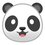# Infinity

I have always thought about how confusing infinity is... Is infinity equal to 0? My proof: Let infinity $= a, a = 2a,$so $0=a$. - Is there something wrong with my proof? -Explain why you chose what you chose for #1.Note by Genius Kid
3 weeks, 1 day ago

This discussion board is a place to discuss our Daily Challenges and the math and science related to those challenges. Explanations are more than just a solution — they should explain the steps and thinking strategies that you used to obtain the solution. Comments should further the discussion of math and science.

When posting on Brilliant:

• Use the emojis to react to an explanation, whether you're congratulating a job well done , or just really confused .
• Ask specific questions about the challenge or the steps in somebody's explanation. Well-posed questions can add a lot to the discussion, but posting "I don't understand!" doesn't help anyone.
• Try to contribute something new to the discussion, whether it is an extension, generalization or other idea related to the challenge.

MarkdownAppears as
*italics* or _italics_ italics
**bold** or __bold__ bold
- bulleted- list
• bulleted
• list
1. numbered2. list
1. numbered
2. list
Note: you must add a full line of space before and after lists for them to show up correctly
paragraph 1paragraph 2

paragraph 1

paragraph 2

[example link](https://brilliant.org)example link
> This is a quote
This is a quote
    # I indented these lines
# 4 spaces, and now they show
# up as a code block.

print "hello world"
# I indented these lines
# 4 spaces, and now they show
# up as a code block.

print "hello world"
MathAppears as
Remember to wrap math in $$ ... $$ or $ ... $ to ensure proper formatting.
2 \times 3 $2 \times 3$
2^{34} $2^{34}$
a_{i-1} $a_{i-1}$
\frac{2}{3} $\frac{2}{3}$
\sqrt{2} $\sqrt{2}$
\sum_{i=1}^3 $\sum_{i=1}^3$
\sin \theta $\sin \theta$
\boxed{123} $\boxed{123}$

Sort by:

I agree with @Zakir Husain. Infinity is not exactly a number. I like to think of infinity as "everything" and zero as "nothing".

However, if we're going to use either infinity or zero as numbers, like when doing calculations with other numbers for instance, we have to have special rules in place which will protect us from making a mistake.

For instance, though many would disagree, I would propose that rule #1 is:

• $\frac{x}{0} = \infty$

where $x$ is any number except $0$.

And because of that condition, rule #2 would be:

• $\frac{0}{0}$ and $\frac{\infty}{\infty}$ are undefined.

Here are the last few rules for working with $0$ and $\infty$. Sometimes $0$ behaves like $\infty$, but other times it does the exact opposite:

• $x + 0 = x$ BUT $x + \infty = \infty$ (adding nothing to $x$ gives $x$, BUT adding everything to $x$ gives everything)

• $x \cdot 0 = 0$ AND $x \cdot \infty = \infty$ (multiplying $x$ and nothing gives nothing AND multiplying $x$ and everything gives everything)

So in your original proof, it is impossible to subtract $a$ ($\infty$) from both sides and have $0$ on one side, and $\infty$ on the other. By rule 4, $2 \cdot \infty = \infty$, so we would be left with $0$ on both sides.

Hope this helps! Sorry if it was too long :)

- 3 weeks ago

Thanks!

- 3 weeks ago

Your welcome! Just keep in mind that those rules are not ones mathematicians have formally agreed upon or anything. They're just basic math combined with my own propositions. :)

- 2 weeks, 5 days ago

- 2 weeks, 5 days ago

Thanks Mei!

- 2 weeks, 4 days ago

Infinity is not a number, it is a concept in mathematics. You can's treat it as a number.

- 3 weeks, 1 day ago

Yes, I agree. Plus this reminds me of the Numberphile video you shared. "types of infinity"

- 3 weeks, 1 day ago

I always pictured infinity as the longest closed curve possible...in the real world of course

- 3 weeks, 1 day ago

I don't think you can apply normal mathematics to infinity, As @Zakir Husain Sir said, $\infty$ is not a number but a concept.

- 2 weeks, 5 days ago